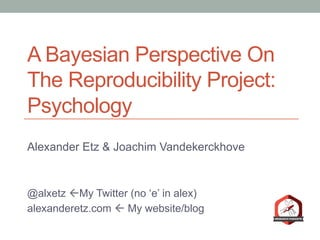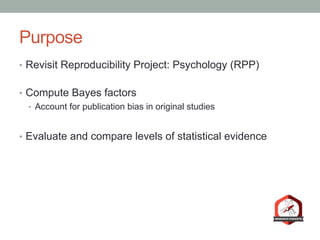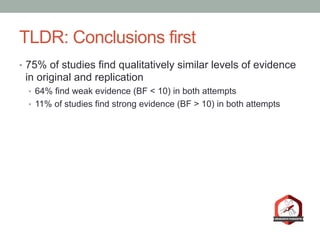Successfully reported this slideshow.

# Bayes rpp bristol×

# Bayes rpp bristol

In this talk I discuss our recent Bayesian reanalysis of the Reproducibility Project: Psychology.

The slides at the end include the technical details underlying the Bayesian model averaging method we employ.

In this talk I discuss our recent Bayesian reanalysis of the Reproducibility Project: Psychology.

The slides at the end include the technical details underlying the Bayesian model averaging method we employ.

### Bayes rpp bristol

1. 1. A Bayesian Perspective On The Reproducibility Project: Psychology Alexander Etz & Joachim Vandekerckhove @alxetz ßMy Twitter (no ‘e’ in alex) alexanderetz.com ß My website/blog
2. 2. Purpose •  Revisit Reproducibility Project: Psychology (RPP) •  Compute Bayes factors •  Account for publication bias in original studies •  Evaluate and compare levels of statistical evidence
3. 3. TLDR: Conclusions first •  75% of studies find qualitatively similar levels of evidence in original and replication •  64% find weak evidence (BF < 10) in both attempts •  11% of studies find strong evidence (BF > 10) in both attempts
4. 4. TLDR: Conclusions first •  75% of studies find qualitatively similar levels of evidence in original and replication •  64% find weak evidence (BF < 10) in both attempts •  11% of studies find strong evidence (BF > 10) in both attempts •  10% find strong evidence in replication but not original •  15% find strong evidence in original but not replication
5. 5. The RPP •  270 scientists attempt to closely replicate 100 psychology studies •  Use original materials (when possible) •  Work with original authors •  Pre-registered to avoid bias •  Analysis plan specified in advance •  Guaranteed to be published regardless of outcome
6. 6. The RPP •  2 main criteria for grading replication
7. 7. The RPP •  2 main criteria for grading replication •  Is the replication result statistically significant (p < .05) in the same direction as original? •  39% success rate
8. 8. The RPP •  2 main criteria for grading replication •  Is the replication result statistically significant (p < .05) in the same direction as original? •  39% success rate •  Does the replication’s confidence interval capture original reported effect? •  47% success rate
9. 9. The RPP •  Neither of these metrics are any good •  (at least not as used) •  Neither make predictions about out-of-sample data •  Comparing significance levels is bad •  “The difference between significant and not significant is not necessarily itself significant” •  -Gelman & Stern (2006)
10. 10. The RPP •  Nevertheless, .51 correlation between original & replication effect sizes •  Indicates at least some level of robustness
11. 11. −0.50 −0.25 0.00 0.25 0.50 0.75 1.00 0.00 0.25 0.50 0.75 1.00 Original Effect Size ReplicationEffectSize p−value Not Significant Significant Replication Power 0.6 0.7 0.8 0.9 Source: Open Science Collaboration. (2015). Estimating the reproducibility of psychological science. Science, 349(6251), aac4716.
12. 12. What can explain the discrepancies?
13. 13. Moderators •  Two study attempts are in different contexts •  Texas vs. California •  Different context = different results? •  Conservative vs. Liberal sample
14. 14. Low power in original studies •  Statistical power: •  The frequency with which a study will yield a statistically significant effect in repeated sampling, assuming that the underlying effect is of a given size. •  Low powered designs undermine credibility of statistically significant results •  Button et al. (2013) •  Type M / Type S errors (Gelman & Carlin, 2014)
15. 15. Low power in original studies •  Replications planned to have minimum 80% power •  Report average power of 92%
16. 16. Publication bias •  Most published results are “positive” findings •  Statistically significant results •  Most studies designed to reject H0 •  Most published studies succeed •  Selective preference = bias •  “Statistical significance filter”
17. 17. Statistical significance filter •  Incentive to have results that reach p < .05 •  “Statistically significant” •  Evidential standard •  Studies with large effect size achieve significance •  Get published
18. 18. Statistical significance filter •  Studies with smaller effect size don’t reach significance •  Get suppressed •  Average effect size inevitably inflates •  Replication power calculations meaningless
19. 19. Can we account for this bias? •  Consider publication as part of data collection process •  This enters through likelihood function •  Data generating process •  Sampling distribution
20. 20. Mitigation of publication bias •  Remember the statistical significance filter •  We try to build a statistical model of it
21. 21. Mitigation of publication bias •  We formally model 4 possible significance filters •  4 models comprise overall H0 •  4 models comprise overall H1 •  If result consistent with bias, then Bayes factor penalized •  Raise the evidence bar
22. 22. Mitigation of publication bias •  Expected distribution of test statistics that make it to the literature.
23. 23. Mitigation of publication bias •  None of these are probably right •  (Definitely all wrong) •  But it is a reasonable start •  Doesn’t matter really •  We’re going to mix and mash them all together •  “Bayesian Model Averaging”
24. 24. The Bayes Factor •  How the data shift the balance of evidence •  Ratio of predictive success of the models BF10 = p(data | H1) p(data | H0 )
25. 25. The Bayes Factor •  H0: Null hypothesis •  H1: Alternative hypothesis BF10 = p(data | H1) p(data | H0 )
26. 26. The Bayes Factor •  BF10 > 1 means evidence favors H1 •  BF10 < 1 means evidence favors H0 •  Need to be clear what H0 and H1 represent BF10 = p(data | H1) p(data | H0 )
27. 27. The Bayes Factor •  H0: d = 0 BF10 = p(data | H1) p(data | H0 )
28. 28. The Bayes Factor •  H0: d = 0 •  H1: d ≠ 0 BF10 = p(data | H1) p(data | H0 )
29. 29. The Bayes Factor •  H0: d = 0 •  H1: d ≠ 0 (BAD) •  Too vague •  Doesn’t make predictions BF10 = p(data | H1) p(data | H0 )
30. 30. The Bayes Factor •  H0: d = 0 •  H1: d ~ Normal(0, 1) •  The effect is probably small •  Almost certainly -2 < d < 2” BF10 = p(data | H1) p(data | H0 )
31. 31. Statistical evidence •  Do independent study attempts obtain similar amounts of evidence?
32. 32. Statistical evidence •  Do independent study attempts obtain similar amounts of evidence? •  Same prior distribution for both attempts •  Measuring general evidential content •  We want to evaluate evidence from outsider perspective
33. 33. Interpreting evidence •  “How convincing would these data be to a neutral observer?” •  1:1 prior odds for H1 vs. H0 •  50% prior probability for each
34. 34. Interpreting evidence •  BF > 10 is sufficiently evidential •  10:1 posterior odds for H1 vs. H0 (or vice versa) •  91% posterior probability for H1 (or vice versa)
35. 35. Interpreting evidence •  BF > 10 is sufficiently evidential •  10:1 posterior odds for H1 vs. H0 (or vice versa) •  91% posterior probability for H1 (or vice versa) •  BF of 3 is too weak •  3:1 posterior odds for H1 vs. H0 (or vice versa) •  Only 75% posterior probability for H1 (or vice versa)
36. 36. Interpreting evidence •  It depends on context (of course) •  You can have higher or lower standards of evidence
37. 37. Interpreting evidence •  How do p values stack up? •  American Statistical Association: •  “Researchers should recognize that a p-value … near 0.05 taken by itself offers only weak evidence against the null hypothesis. “
38. 38. Interpreting evidence •  How do p values stack up? •  p < .05 is weak standard •  p = .05 corresponds to BF ≤ 2.5 (at BEST) •  p = .01 corresponds to BF ≤ 8 (at BEST)
39. 39. Face-value BFs •  Standard Bayes factor •  Bias free •  Results taken at face-value
40. 40. Bias-mitigated BFs •  Bayes factor accounting for possible bias
41. 41. Illustrative Bayes factors •  Study 27 •  t(31) = 2.27, p=.03 •  Maximum BF10 = 3.4
42. 42. Illustrative Bayes factors •  Study 27 •  t(31) = 2.27, p=.03 •  Maximum BF10 = 3.4 •  Face-value BF10 = 2.9
43. 43. Illustrative Bayes factors •  Study 27 •  t(31) = 2.27, p=.03 •  Maximum BF10 = 3.4 •  Face-value BF10 = 2.9 •  Bias-mitigated BF10 = .81
44. 44. Illustrative Bayes factors •  Study 71 •  t(373) = 4.4, p < .001 •  Maximum BF10 = ~2300
45. 45. Illustrative Bayes factors •  Study 71 •  t(373) = 4.4, p < .001 •  Maximum BF10 = ~2300 •  Face-value BF10 = 947
46. 46. Illustrative Bayes factors •  Study 71 •  t(373) = 4.4, p < .001 •  Maximum BF10 = ~2300 •  Face-value BF10 = 947 •  Bias-mitigated BF10 = 142
47. 47. RPP Sample •  N=72 •  All univariate tests (t test, anova w/ 1 model df, etc.)
48. 48. Results •  Original studies, face-value •  Ignoring pub bias •  43% obtain BF10 > 10 •  57% obtain 1/10 < BF10 < 10 •  0 obtain BF10 < 1/10
49. 49. Results •  Original studies, bias-corrected •  26% obtain BF10 > 10 •  74% obtain 1/10 < BF10 < 10 •  0 obtain BF10 < 1/10
50. 50. Results •  Replication studies, face value •  No chance for bias, no need for correction •  21% obtain BF10 > 10 •  79% obtain 1/10 < BF10 < 10 •  0 obtain BF10 < 1/10
51. 51. Consistency of results •  No alarming inconsistencies •  46 cases where both original and replication show only weak evidence •  Only 8 cases where both show BF10 > 10
52. 52. Consistency of results •  11 cases where original BF10 > 10, but not replication •  7 cases where replication BF10 > 10, but not original •  In every case, the study obtaining strong evidence had the larger sample size
53. 53. Moderators? •  As Laplace would say, we have no need for that hypothesis •  Results adequately explained by: •  Publication bias in original studies •  Generally weak standards of evidence
54. 54. Take home message •  Recalibrate our intuitions about statistical evidence •  Reevaluate expectations for replications •  Given weak evidence in original studies
55. 55. Thank you
56. 56. Thank you @alxetz ßMy Twitter (no ‘e’ in alex) alexanderetz.com ß My website/blog @VandekerckhoveJ ß Joachim’s Twitter joachim.cidlab.com ß Joachim’s website
57. 57. Want to learn more Bayes? •  My blog: •  alexanderetz.com/understanding-bayes •  “How to become a Bayesian in eight easy steps” •  http://tinyurl.com/eightstepsdraft •  JASP summer workshop •  https://jasp-stats.org/ •  Amsterdam, August 22-23, 2016
58. 58. Technical Appendix
59. 59. Mitigation of publication bias •  Model 1: No bias •  Every study has same chance of publication •  Regular t distributions •  H0 true: Central t •  H0 false: Noncentral t •  These are used in standard BFs
60. 60. Mitigation of publication bias •  Model 2: Extreme bias •  Only statistically significant results published •  t distributions but… •  Zero density in the middle •  Spikes in significant regions
61. 61. Mitigation of publication bias •  Model 3: Constant-bias •  Nonsignificant results published x% as often as significant results •  t distributions but… •  Central regions downweighted •  Large spikes over significance regions
62. 62. Mitigation of publication bias •  Model 4: Exponential bias •  “Marginally significant” results have a chance to be published •  Harder as p gets larger •  t likelihoods but… •  Spikes over significance regions •  Quick decay to zero density as (p – α) increases
63. 63. Calculate face-value BF •  Take the likelihood: •  Integrate with respect to prior distribution, p(δ) tn (x |δ)
64. 64. Calculate face-value BF •  Take the likelihood: •  Integrate with respect to prior distribution, p(δ) •  “What is the average likelihood of the data given this model?” •  Result is marginal likelihood, M tn (x |δ)
65. 65. Calculate face-value BF •  For H1: δ ~ Normal(0 , 1) •  For H0: δ = 0 M− = tn (x |δ = 0) M+ = tn (x |δ) Δ ∫ p(δ)dδ
66. 66. Calculate face-value BF •  For H1: δ ~ Normal(0 , 1) •  For H0: δ = 0 M− = tn (x |δ = 0) M+ = tn (x |δ) Δ ∫ p(δ)dδ BF10= p(data | H1) = M+ p(data | H0 ) = M−
67. 67. Calculate mitigated BF •  Start with regular t likelihood function •  Multiply it by bias function: w = {1, 2, 3, 4} •  Where w=1 is no bias, w=2 is extreme bias, etc. tn (x |δ)
68. 68. Calculate mitigated BF •  Start with regular t likelihood function •  Multiply it by bias function: w = {1, 2, 3, 4} •  Where w=1 is no bias, w=2 is extreme bias, etc. •  E.g., when w=3 (constant bias): tn (x |δ) tn (x |δ)× w(x |θ)
69. 69. Calculate mitigated BF •  Too messy •  Rewrite as a new function
70. 70. Calculate mitigated BF •  Too messy •  Rewrite as a new function •  H1: δ ~ Normal(0 , 1): pw+ (x | n,δ,θ)∝tn (x |δ)× w(x |θ)
71. 71. Calculate mitigated BF •  Too messy •  Rewrite as a new function •  H1: δ ~ Normal(0 , 1): •  H0: δ = 0: pw+ (x | n,δ,θ)∝tn (x |δ)× w(x |θ) pw− (x | n,θ) = pw+ (x | n,δ = 0,θ)
72. 72. Calculate mitigated BF •  Integrate w.r.t. p(θ) and p(δ) •  “What is the average likelihood of the data given each bias model?” •  p(data | bias model w):
73. 73. Calculate mitigated BF •  Integrate w.r.t. p(θ) and p(δ) •  “What is the average likelihood of the data given each bias model?” •  p(data | bias model w): Mw+ = Δ ∫ pw+ (x | n,δ,θ) Θ ∫ p(δ)p(θ)dδdθ
74. 74. Calculate mitigated BF •  Integrate w.r.t. p(θ) and p(δ) •  “What is the average likelihood of the data given each bias model?” •  p(data | bias model w): Mw+ = Δ ∫ pw+ (x | n,δ,θ) Θ ∫ p(δ)p(θ)dδdθ Mw− = pw− (x | n,θ) Θ ∫ p(θ)dθ
75. 75. Calculate mitigated BF •  Take Mw+ and Mw- and multiply by the weights of the corresponding bias model, then sum within each hypothesis
76. 76. Calculate mitigated BF •  Take Mw+ and Mw- and multiply by the weights of the corresponding bias model, then sum within each hypothesis •  For H1: p(w =1)M1+ + p(w = 2)M2+ + p(w = 3)M3+ + p(w = 4)M4+
77. 77. Calculate mitigated BF •  Take Mw+ and Mw- and multiply by the weights of the corresponding bias model, then sum within each hypothesis •  For H1: •  For H0: p(w =1)M1+ + p(w = 2)M2+ + p(w = 3)M3+ + p(w = 4)M4+ p(w =1)M1− + p(w = 2)M2− + p(w = 3)M3− + p(w = 4)M4−
78. 78. Calculate mitigated BF •  Messy, so we restate as sums:
79. 79. Calculate mitigated BF •  Messy, so we restate as sums: •  For H1: w ∑ p(w)Mw+
80. 80. Calculate mitigated BF •  Messy, so we restate as sums: •  For H1: •  For H0: w ∑ p(w)Mw+ w ∑ p(w)Mw−
81. 81. Calculate mitigated BF •  Messy, so we restate as sums: •  For H1: BF10 = •  For H0: w ∑ p(w)Mw+ w ∑ p(w)Mw− p(data | H1) = w ∑ p(w)Mw+ p(data | H0 ) = w ∑ p(w)Mw−